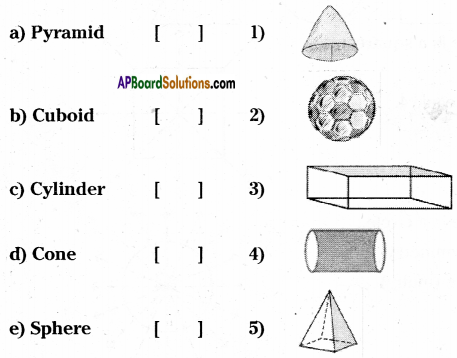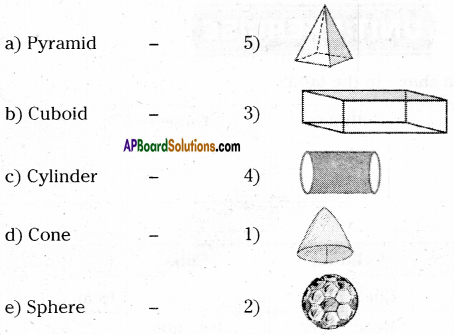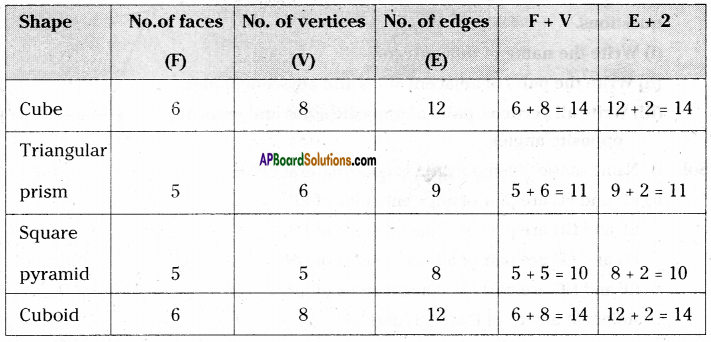AP State Syllabus AP Board 6th Class Maths Solutions Chapter 9 2D-3D Shapes Ex 9.4 Textbook Questions and Answers.

## AP State Syllabus 6th Class Maths Solutions 9th Lesson 2D-3D Shapes Ex 9.4Question 1.
Write the shapes of the following.
(i) A brick (ii) A road roller (iii) Foot ball (iv) Joker cap
Solution:
i) Shape of a brick is cuboid.
ii) Shape of a road roller is cylinder.
iii) Shape of a foot ball is shpere.
iv) Shape of a joker cap is cone.

Question 2.
Fill in the blanks.
(i) The shape of heap of grain
(ii) The shape of a dice
(iii) The shape of a bubble
(iv) The shape of a candle
Solution:
i) Cone ii) Cube iii) Sphere

Question 3.
Match the following.Solution:Question 4.
Fill in the table.

 Shape No.of faces No. of vertices No. of edges Cube Triangular prism Square pyramid Cuboid

Verify Euler’s Formula for the data in the table.We know that, the F + V = E + 2is Euler’s formula.
In the above table given shapes satisfy the Euler’s formula.